# Slope Of A Line (10 Common Questions Answered)

Slope is a useful concept in algebra, calculus, and in the sciences.  It can be applied to many fields outside of STEM as well, so it helps to know about slope.

So, what do you need to know about the slope of a line?  The slope of a line can be positive, negative, zero, or undefined. A slope can be a whole number, fraction, mixed number, or decimal. Slope is a rate of change whose units can help to interpret this rate. The slope-intercept & point-slope forms are both valid to describe a line with an equation.

Of course, we can also compare the slopes (and y-intercepts) of any two lines to tell if they are parallel, perpendicular, or the same line (or perhaps none of these three possibilities).

In this article, we’ll talk about the slope of a line and answer some common questions about it.  We’ll also talk about line forms and go through some examples to make the concept clear.

Let’s get started.

## Slope Of A Line

The slope of a line tells us the direction and steepness of the line.  For a line with the equation y = mx + b, m is the slope and b is the y-intercept.

The slope is often written in words as “slope equals rise over run”, where rise is the vertical change (y variable) and run is the horizontal change (x variable).

In symbols, the slope m is given by:

• m = (y2 – y1) / (x2 – x1)

When using slope to determine direction:

• If slope is positive, then the line moves up as we move right (y increases as x increases).
• If slope is negative, then the line moves down as we move right (y decreases as x increases).
• If slope is zero, then the line moves neither up nor down as we move right (y is unchanged as x increases).  This is a horizontal line.
• If slope is undefined, then the line moves neither left nor right as we move right (x is unchanged as y increases or decreases).  This is a vertical line.

The table below summarizes what slope means for different types of lines.

When using slope to determine steepness:

• The larger the absolute value of the slope, the steeper the line.
• A slope of 0 is like flat ground.
• A slope of 1 is like a roof slanted at a 45 degree angle.

The slope of a line is also the tangent of the angle formed by the x-axis and the line (pictured below).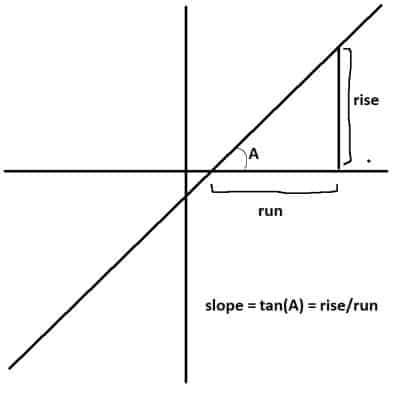The slope of a line is the rise over run, or the tangent of the angle A formed by the line and the x-axis.

### Can Slope Be Zero?

Slope can be zero in the case of a horizontal line.  For a line equation y = mx + b, a slope of zero means m = 0, which gives us the simplified equation y = b.

The value of b will tell us the value of y for any x-value we choose (a horizontal line is a constant function).

Another way to think about zero slope is a zero “rise” in the “slope equals rise over run” equation (since y never changes, there is always a zero rise):

• slope = rise / run
• slope = 0 / run
• slope = 0

The graph below shows a horizontal line, which has a slope of zero.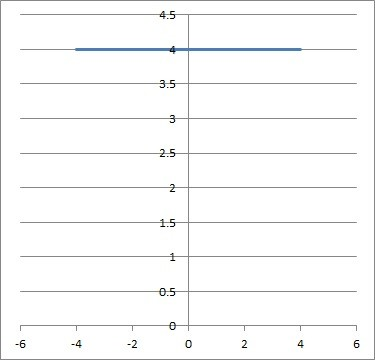This is the graph of the horizontal line y = 4, which has a slope of m = 0.

### Can Slope Be Negative?

A slope can be negative – slope is not always positive.  For a line equation y = mx + b, a negative slope means m < 0.

A slope is negative when the “rise” and “run” parts of the slope equation have opposite signs.

The table below shows how the sign of rise and run affect the sign of the slope.

A line with a negative slope moves from the “top left” of a graph towards the “bottom right” of a graph.

The graph below shows a line with a negative slope.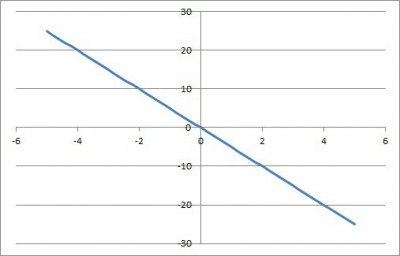The line y = -5x has a negative slope of m = -5. It also happens to have a y-intercept of zero: b = 0.

### Can Slope Be A Fraction?

A slope can be a fraction – in fact, any slope can be written as a fraction.  For example, a slope that is a whole number (like m = 2 or m = -5) can be written as a fraction (m = 2/1 or m = -5/1).

Remember that slope is defined as “rise over run”, or the ratio of the change in y over the change in x.  A ratio always gives us a fraction, but in some cases we can simplify to a whole number.

#### Example: A Slope That Is A Fraction

For the line y = (-8/5)x – 10, the slope is m = -8/5, which is a fraction.  It cannot be simplified to a whole number.

You can see the graph of this line pictured below.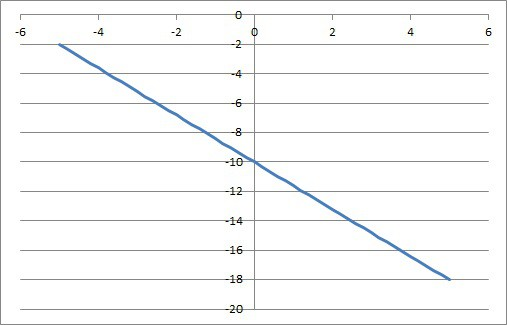The line y = (-8/5)x – 10 has a slope that is a fraction: b = -8/5.

### Can Slope Be A Mixed Number?

A slope can be a mixed number if we want to express it that way (instead of as an improper fraction).  Consider the example above: the line y = (-8/5)x – 10.

The slope is m = -8/5, but we can convert the improper fraction -8/5 to the mixed number -1 & 3/5.

No matter how we express the slope (improper fraction or mixed number), we still get the same line on a graph.

### Can Slope Be A Decimal?

A slope can be a decimal – in fact, any slope can be written as a decimal.  For example, a slope that is a whole number (like m = 2 or m = -5) can be written as a decimal (m = 2.0 or m = -5.0).

Remember that slope is defined as “rise over run”, or the ratio of the change in y over the change in x.  A ratio always gives us a fraction, but we can always convert that fraction to a decimal.

Note: in some cases, the decimal will be repeating.  For example, 1/3 = 0.3333….

#### Example: A Slope That Is A Decimal

For the line y = -2.75x + 3, the slope is m = -2.75, which is a decimal.  It cannot be simplified to a whole number.

You can see the graph of this line pictured below.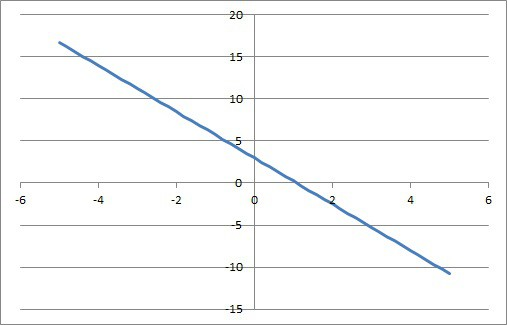Here is the graph of the line y = -2.75x + 3, which has a decimal slope: m = -2.75.

### Can Slope Be Undefined?

Slope can be undefined in the case of a vertical line.  For a line equation y = mx + b, an undefined slope means a zero denominator for m, and we have the simplified equation x = a.

The value of a will tell us the value of x for any y-value we choose (a vertical line is not a function, since it fails the vertical line test).

Another way to think about undefined slope is a zero “run” in the “slope equals rise over run” equation (since x never changes, there is always a zero run):

• slope = rise / run
• slope = rise / 0
• slope = undefined

The graph below shows a vertical line, which has an undefined slope.

### Does Slope Have Units?

Slope has units according to the units for the x and y variables.  The units for slope are: “units of y per unit of x”.

For example, let’s say x has units of “hours” and y has units of “miles”.  Then the units for slope would be “units of y per unit of x”, or “miles per hour”.

This slope is really a speed, which is telling us how fast an object is moving.Units can help us to interpret what slope is telling us. For example, miles per hour is telling us about the speed of an object.

If you traveled at a constant speed and covered 40 miles in 2 hours, then your speed would be 40 miles / 2 hours = 20 miles per hour.

The units of slope can help you to interpret what the slope is telling you.  So, pay attention to the axis labels for a graph to determine the units.

### Are Slope & Rate Of Change The Same?

Slope and rate of change are the same thing.  A slope is “rise over run”, which is really “change in y divided by change in x”.

The rate of change is really referring to “rate of change of y, with respect to x”.  In other words, rate of change answers the question: “how much is y changing when we increase x by one units?”

The speed of 20 miles per hour (from the previous section) tells us the rate of change of the total distance we have traveled with respect to time.

## Line Forms

There are three basic forms of a line equation:

• Standard Form
• Slope-Intercept Form
• Point-Slope Form

Each one has a slightly different appearance, and each is solved for a different value, variable, or expression.

Let’s take a closer look at these three forms, starting with standard form.

### What Is Standard Form Of A Line?

The standard form of a line is Ax + By = C, where A, B, and C are constants.

Note:

• If A = 0, we get the horizontal line By = C (or y = C/B).
• If B = 0, we get the vertical line Ax = C (or x = C/A).

We cannot have both A and B zero, or else we would not have a line at all.

We can solve the standard form for y to get slope-intercept form:

• Ax + By = C
• By = -Ax + C
• y = (-A/B)x + (C/B)

The slope is –A/B and the y-intercept is C/B.

### What Is Slope Intercept Form?

The slope-intercept form of a line is y = mx + b, where m and b are constants.  The value of m is the slope, and the value of b is the y-intercept.

Note:

• If b = 0, we get the line y = mx, which goes through the origin (0, 0).
• If m = 0, we get the horizontal line y = b.
• If both m = 0 and b = 0, we get the horizontal line y = 0.
• If m is undefined, we get the vertical line x = a.

We can rearrange the slope-intercept form to get standard form:

• y = mx + b
• -mx + 1y = b

In this case, the coefficients for standard form are A = -m, B = 1, and C = b.

### What Is Point Slope Form?

The point-slope form of a line is y – y1 = m(x – x1), where m, x1, and x2, are constants.  The value of m is the slope, and the values of x1 and y1 give us a point (x1, y1) on the line.

Note:

• If m = 0, we get the horizontal line y = y1.

We can solve the point-slope form for y to get slope-intercept form:

• y – y1 = m(x – x1)
• y = m(x – x1) + y1
• y = mx – mx1 + y1
• y = mx + (y1 – mx1)

In this case, the coefficients for slope-intercept form are m = m and b = y1 – mx1.

## Conclusion

Now you know a little more about the slope of a line, what it means, and how to calculate it.  You also know what form the slope of a line can take.